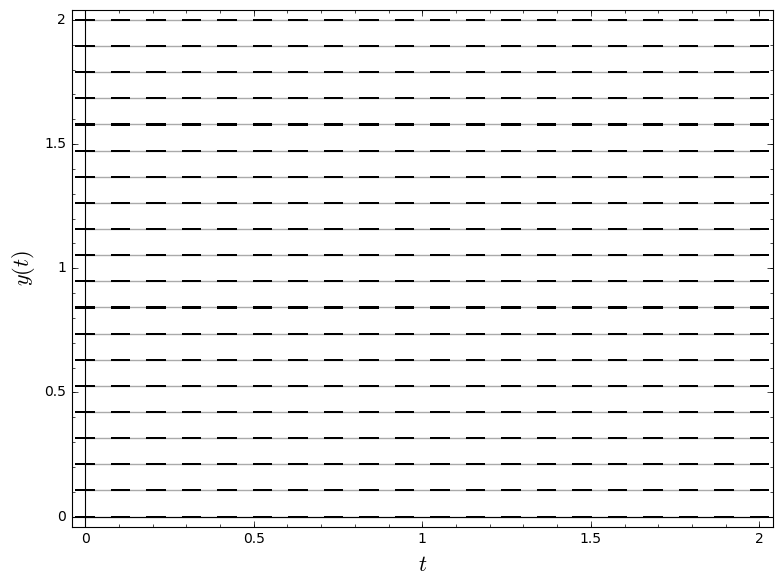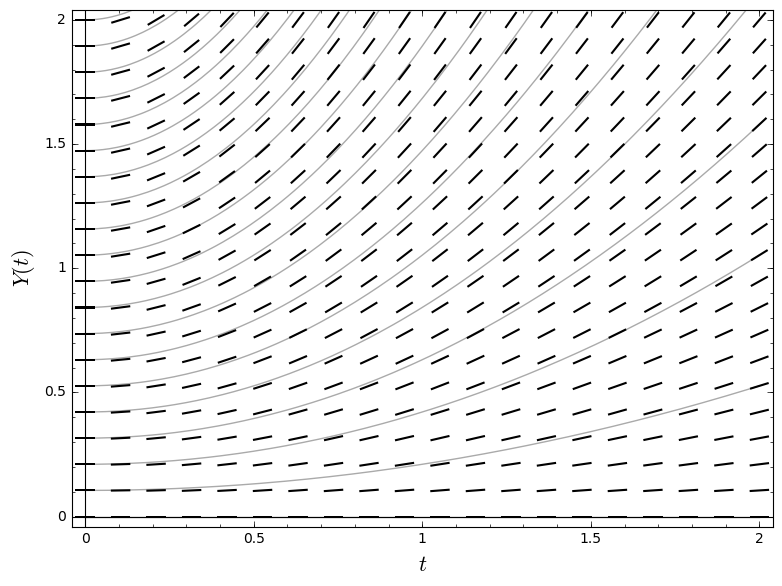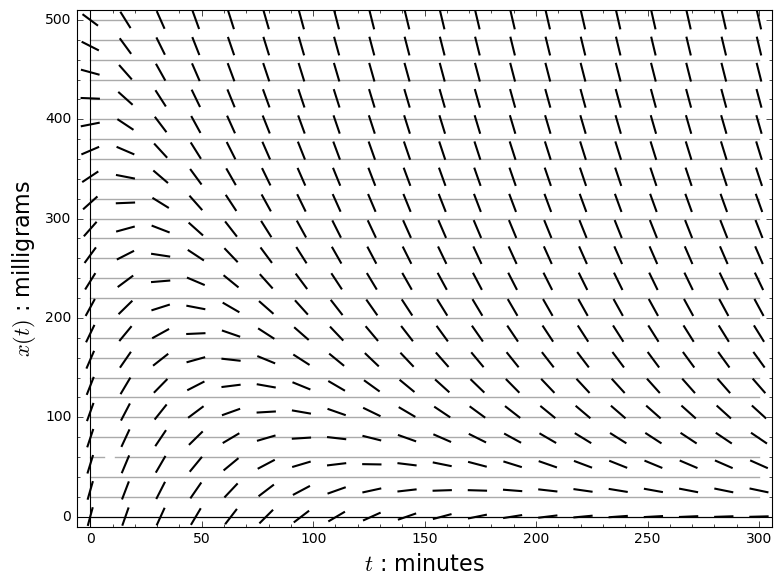# Rescaling the unknown function¶

Or, if you want to sound fancy, "the method of integrating factors"

In :
t, x, X, y, Y = var('t x X y Y')
palette = [(215/255, 0/255, 132/255), (255/255, 1/255, 73/255), (255/255, 121/255, 1/255), (255/255, 210/255, 0/255)]
cool_palette = [(0/255, 150/255, 173/255), (0/255, 200/255, 146/255)]
grid_gray = (2/3, 2/3, 2/3)


## The dumbest differential equation¶

### The original equation¶

$$y'(t) = 0$$

In :
const_grid = sum([plot((2/19)*k, (t, 0, 2), rgbcolor = grid_gray, zorder = 0) for k in range(20)])
const_direction = plot_slope_field(0, (t, 0, 2), (y, 0, 2))
const_labels = ["$t$", "$y(t)$"]
show(const_direction + const_grid, axes_labels = const_labels)### The equation describing the rescaled function¶

\begin{align*} Y(t) & = (1 + t^2)\,y(t) \\ Y'(t) & = 2t\,y(t) + (1 + t^2)\,y'(t) \\ & = \frac{2t}{1 + t^2}\,Y(t) \end{align*}

In :
scaled_const_grid = sum([plot((1 + t^2) * (2/19)*k, (t, 0, 2), rgbcolor = grid_gray, ymax = 2, zorder = 0) for k in range(20)])
scaled_const_direction = plot_slope_field(2*t/(1+t^2) * Y, (t, 0, 2), (Y, 0, 2))
scaled_const_labels = ["$t$", "$Y(t)$"]
show(scaled_const_direction + scaled_const_grid, axes_labels = scaled_const_labels)## Administering an antibiotic by pill¶

In :
blood_grid = sum([plot(k*20, (t, 0, 300), rgbcolor = grid_gray, zorder = 0) for k in range(26)])
blood_direction = plot_slope_field((1/60)*500*exp(-t/60) - (1/50)*x, (t, 0, 300), (x, 0, 500))
blood_labels = ["$t$ : minutes", "$x(t)$ : milligrams"]
show(blood_direction + blood_grid, axes_labels = blood_labels)In :
scaled_blood_grid = sum([plot((60/500)*exp(t/50)*(k*20), (t, 0, 300), ymax = 500, rgbcolor = grid_gray, zorder = 0) for k in range(211)])
scaled_blood_direction = plot_slope_field(exp((1/50 - 1/60)*t), (t, 0, 300), (X, 0, 500))
scaled_blood_solution = plot(300*(exp((1/50 - 1/60)*t) - 1), (t, 0, 300), thickness = 3, rgbcolor = cool_palette)
scaled_blood_labels = ["$t$ : minutes", "$X(t)$"]
show(scaled_blood_direction + scaled_blood_grid + scaled_blood_solution, axes_labels = scaled_blood_labels)